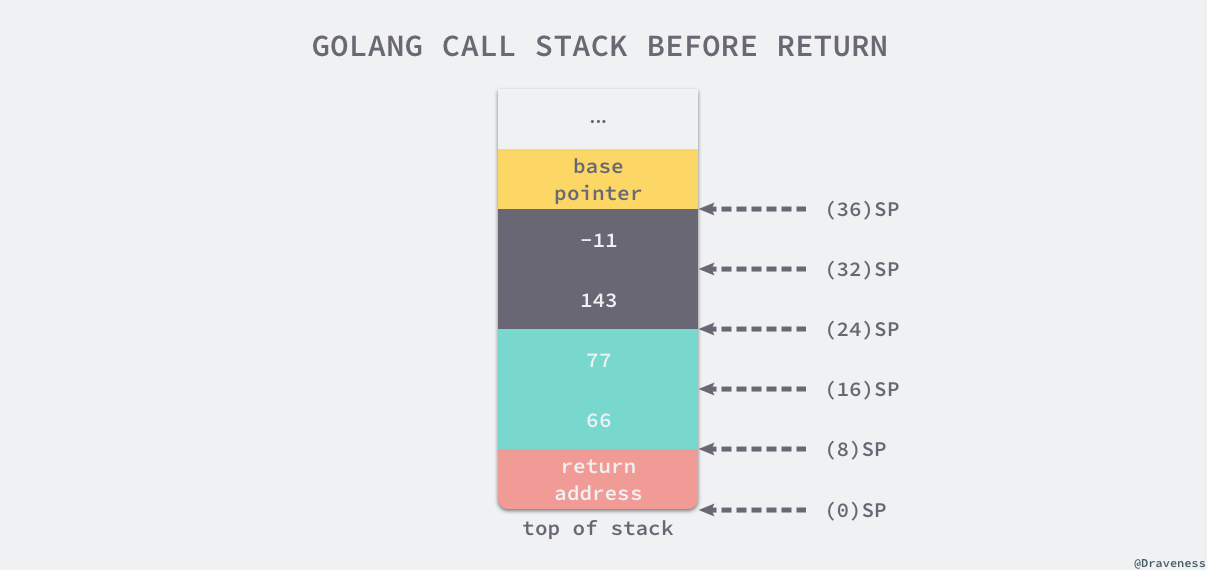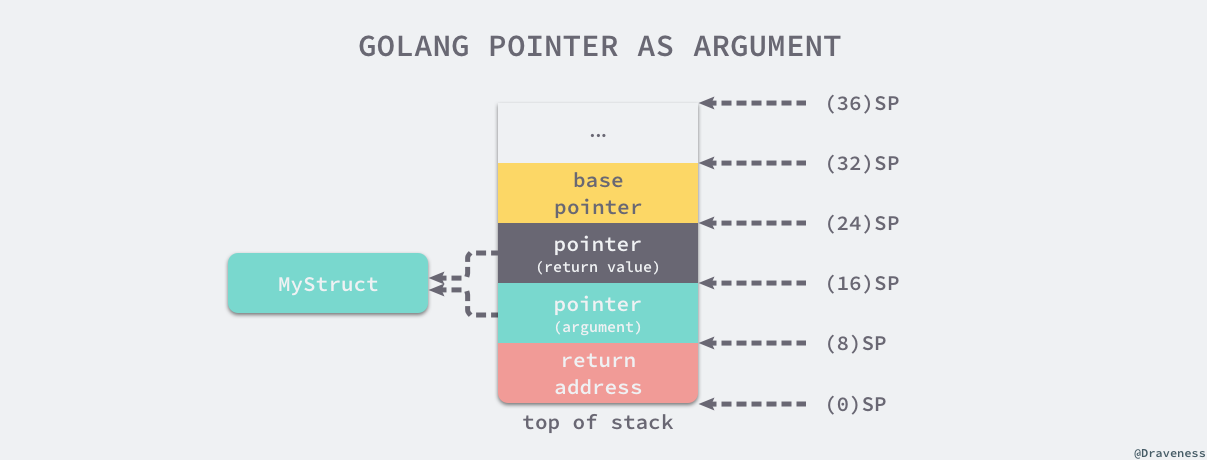2.1 函数调用

1. 调用惯例

somefunction(arg0, arg1)

1.1. C 语言

\$ gcc --version
gcc (Ubuntu 4.8.2-19ubuntu1) 4.8.2
Copyright (C) 2013 Free Software Foundation, Inc.
This is free software; see the source for copying conditions.  There is NO
warranty; not even for MERCHANTABILITY or FITNESS FOR A PARTICULAR PURPOSE.

\$ uname -a
Linux iZ255w13cy6Z 3.13.0-32-generic #57-Ubuntu SMP Tue Jul 15 03:51:08 UTC 2014 x86_64 x86_64 x86_64 GNU/Linux

gcc 和 clang 虽然在编译 C 语言代码时生成的汇编语言可能有比较大的差别，但是生成代码的结构不会有太大的区别，需要注意的是不同内核版本的操作系统生成的汇编指令可能有比较大的不同，不过对于我们这些只是想要了解实现原理的开发者来说没有太多的影响。

int my_function(int arg1, int arg2) {
return arg1 + arg2;
}

int main() {
int i = my_function(1, 2);
}

my_function:
.LFB0:
.cfi_startproc
pushq    %rbp
.cfi_def_cfa_offset 16
.cfi_offset 6, -16
movq    %rsp, %rbp
.cfi_def_cfa_register 6
movl    %edi, -4(%rbp)
movl    %esi, -8(%rbp)
movl    -8(%rbp), %eax
movl    -4(%rbp), %edx
addl    %edx, %eax // arg1 + arg2
popq    %rbp
.cfi_def_cfa 7, 8
ret
.cfi_endproc
main:
.LFB1:
.cfi_startproc
pushq    %rbp
.cfi_def_cfa_offset 16
.cfi_offset 6, -16
movq    %rsp, %rbp
.cfi_def_cfa_register 6
subq    \$16, %rsp
movl    \$2, %esi // 处理第二个参数
movl    \$1, %edi // 处理第一个参数
call    my_function
movl    %eax, -4(%rbp)
leave
.cfi_def_cfa 7, 8
ret
.cfi_endproc

// main.c
int my_function(int arg1, int arg2, int arg3, int arg4, int arg5, int arg6, int arg7, int arg8) {
return arg1 + arg2 + arg3 + arg4 + arg5 + arg6 + arg7 + arg8;
}

int main() {
my_function(1, 2, 3, 4, 5, 6, 7, 8);
}

// main.s
main:
.LFB1:
.cfi_startproc
pushq    %rbp
.cfi_def_cfa_offset 16
.cfi_offset 6, -16
movq    %rsp, %rbp
.cfi_def_cfa_register 6
subq    \$16, %rsp
movl    \$8, 8(%rsp)
movl    \$7, (%rsp)
movl    \$6, %r9d
movl    \$5, %r8d
movl    \$4, %ecx
movl    \$3, %edx
movl    \$2, %esi
movl    \$1, %edi
call    my_function
leave
.cfi_def_cfa 7, 8
ret
.cfi_endprocmy_function:
.LFB0:
.cfi_startproc
pushq    %rbp
.cfi_def_cfa_offset 16
.cfi_offset 6, -16
movq    %rsp, %rbp
.cfi_def_cfa_register 6
movl    %edi, -4(%rbp)
movl    %esi, -8(%rbp)
movl    %edx, -12(%rbp)
movl    %ecx, -16(%rbp)
movl    %r8d, -20(%rbp)
movl    %r9d, -24(%rbp)
movl    -8(%rbp), %eax // eax = 2
movl    -4(%rbp), %edx // edx = 1
addl    %eax, %edx     // edx = eax + edx = 3
movl    -12(%rbp), %eax
movl    -16(%rbp), %eax
movl    -20(%rbp), %eax
movl    -24(%rbp), %eax
movl    16(%rbp), %eax  // eax = 7
addl    %eax, %edx      // edx = eax + edx = 28
movl    24(%rbp), %eax  // eax = 8
addl    %edx, %eax      // edx = eax + edx = 36
popq    %rbp
.cfi_def_cfa 7, 8
ret
.cfi_endproc

1.2. Golang

package main

func myFunction(a, b int) (int, int) {
return a + b, a - b
}

func main() {
myFunction(66, 77)
}

"".main STEXT size=68 args=0x0 locals=0x28
0x0000 00000 (main.go:7)    TEXT    "".main(SB), \$40-0
0x0000 00000 (main.go:7)    MOVQ    (TLS), CX
0x0009 00009 (main.go:7)    CMPQ    SP, 16(CX)
0x000d 00013 (main.go:7)    JLS    61
0x000f 00015 (main.go:7)    SUBQ    \$40, SP // 分配 40 字节栈空间
0x0013 00019 (main.go:7)    MOVQ    BP, 32(SP) // 将基址指针存储到栈上
0x0018 00024 (main.go:7)    LEAQ    32(SP), BP
0x001d 00029 (main.go:8)    MOVQ    \$66, (SP)  // 第一个参数
0x0025 00037 (main.go:8)    MOVQ    \$77, 8(SP) // 第二个参数
0x002e 00046 (main.go:8)    PCDATA    \$0, \$0
0x002e 00046 (main.go:8)    CALL    "".myFunction(SB)
0x0033 00051 (main.go:9)    MOVQ    32(SP), BP
0x0038 00056 (main.go:9)    ADDQ    \$40, SP
0x003c 00060 (main.go:9)    RETmain 函数中，通过 SUBQ \$40, SP 指令一共在栈上分配了 40 字节的内存空间，最开始的 8 个字节存储了 main 函数的栈基址指针，之后的 16 个字节是为函数 myFunction 的两个返回值预留的空间，最后的 16 字节存储了该函数调用时需要的两个参数，压栈的顺序和 C 语言中一样，也是从右到左。

"".myFunction STEXT nosplit size=49 args=0x20 locals=0x0
0x0000 00000 (main.go:3)    TEXT    "".myFunction(SB), NOSPLIT, \$0-32
0x0000 00000 (main.go:3)    MOVQ    \$0, "".~r2+24(SP) // 初始化第一个返回值
0x0009 00009 (main.go:3)    MOVQ    \$0, "".~r3+32(SP) // 初始化第二个返回值
0x0012 00018 (main.go:4)    MOVQ    "".a+8(SP), AX    // AX = 66
0x0017 00023 (main.go:4)    ADDQ    "".b+16(SP), AX   // AX = AX + 77 = 143
0x001c 00028 (main.go:4)    MOVQ    AX, "".~r2+24(SP) // (24)SP = AX = 143
0x0021 00033 (main.go:4)    MOVQ    "".a+8(SP), AX    // AX = 66
0x0026 00038 (main.go:4)    SUBQ    "".b+16(SP), AX   // AX = AX - 77 = -11
0x002b 00043 (main.go:4)    MOVQ    AX, "".~r3+32(SP) // (32)SP = AX = -11
0x0030 00048 (main.go:4)    RETmyFunction 函数返回之后，main 函数就会通过以下的指令来恢复栈基址指针并销毁已经失去作用的 40 字节的栈空间：

0x0033 00051 (main.go:9)    MOVQ    32(SP), BP
0x0038 00056 (main.go:9)    ADDQ    \$40, SP
0x003c 00060 (main.go:9)    RET

1. 能够降低实现的复杂度；
• 不需要考虑超过寄存器个数的参数应该如何传递；
2. 更方便的兼容不同的硬件；
• 不同 CPU 的寄存器差别比较大；
3. 函数可以具有多个返回值；
• 栈上的内存地址与相比寄存器的个数是无限的；
• 使用寄存器支持多个返回值也会非常困难，超出寄存器个数的返回值也需要使用栈来传递；

2. 参数传递

2.1. 整型和数组

func myFunction(i int, arr int) {
fmt.Printf("in my_funciton - i=%p arr=%p\n", &i, &arr)
}

func main() {
i := 30
arr := int{66, 77}
fmt.Printf("before calling - i=%p arr=%p\n", &i, &arr)
myFunction(i, arr)
fmt.Printf("after  calling - i=%p arr=%p\n", &i, &arr)
}

\$ go run main.go
before calling - i=0xc00009a000 arr=0xc00009a010
in my_funciton - i=0xc00009a008 arr=0xc00009a020
after  calling - i=0xc00009a000 arr=0xc00009a010

func myFunction(i int, arr int) {
i = 29
arr = 88
fmt.Printf("in my_funciton - i=(%d, %p) arr=(%v, %p)\n", i, &i, arr, &arr)
}

func main() {
i := 30
arr := int{66, 77}
fmt.Printf("before calling - i=(%d, %p) arr=(%v, %p)\n", i, &i, arr, &arr)
myFunction(i, arr)
fmt.Printf("after  calling - i=(%d, %p) arr=(%v, %p)\n", i, &i, arr, &arr)
}

\$ go run main.go
before calling - i=(30, 0xc000072008) arr=([66 77], 0xc000072010)
in my_funciton - i=(29, 0xc000072028) arr=([66 88], 0xc000072040)
after  calling - i=(30, 0xc000072008) arr=([66 77], 0xc000072010)

2.2. 结构体和指针

type MyStruct struct {
i int
}

func myFunction(a MyStruct, b *MyStruct) {
a.i = 31
b.i = 41
fmt.Printf("in my_function - a=(%d, %p) b=(%v, %p)\n", a, &a, b, &b)
}

func main() {
a := MyStruct{i: 30}
b := &MyStruct{i: 40}
fmt.Printf("before calling - a=(%d, %p) b=(%v, %p)\n", a, &a, b, &b)
myFunction(a, b)
fmt.Printf("after calling  - a=(%d, %p) b=(%v, %p)\n", a, &a, b, &b)
}

\$ go run main.go
before calling - a=({30}, 0xc000018178) b=(&{40}, 0xc00000c028)
in my_function - a=({31}, 0xc000018198) b=(&{41}, 0xc00000c038)
after calling  - a=({30}, 0xc000018178) b=(&{41}, 0xc00000c028)

package main

import "unsafe"
import "fmt"

type MyStruct struct {
i int
j int
}

func myFunction(ms *MyStruct) {
ptr := unsafe.Pointer(ms)
for i := 0; i < 2; i++ {
c := (*int)(unsafe.Pointer((uintptr(ptr) + uintptr(8*i))))
*c += i + 1
fmt.Printf("[%p] %d\n", c, *c)
}
}

func main() {
a := &MyStruct{i: 40, j: 50}
myFunction(a)
fmt.Printf("[%p] %v\n", a, a)
}

\$ go run main.go
[0xc000018180] 41
[0xc000018188] 52
[0xc000018180] &{41 52}

type MyStruct struct {
i int
j int
}

func myFunction(ms *MyStruct) *MyStruct {
return ms
}

// assembly
"".myFunction STEXT nosplit size=20 args=0x10 locals=0x0
0x0000 00000 (main.go:8)    TEXT    "".myFunction(SB), NOSPLIT, \$0-16
0x0000 00000 (main.go:8)    FUNCDATA    \$0, gclocals·aef1f7ba6e2630c93a51843d99f5a28a(SB)
0x0000 00000 (main.go:8)    FUNCDATA    \$1, gclocals·33cdeccccebe80329f1fdbee7f5874cb(SB)
0x0000 00000 (main.go:8)    MOVQ    \$0, "".~r1+16(SP) // 初始化返回值
0x0009 00009 (main.go:9)    MOVQ    "".ms+8(SP), AX   // 复制引用
0x000e 00014 (main.go:9)    MOVQ    AX, "".~r1+16(SP) // 返回引用
0x0013 00019 (main.go:9)    RET3. 总结

1. 通过堆栈传递参数，入栈的顺序是从右到左；
2. 函数返回值通过堆栈传递并由调用者预先分配内存空间；
3. 调用函数时都是传值，接收方会对入参进行复制再计算；

5. 其他

5.2. 微信公众号